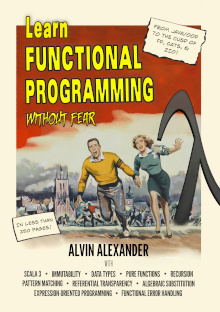# Appendix: Scala `for` expression translation examples

[toc]

This appendix for my book, Functional Programming, Simplified (Scala edition), consists of examples of how different `for` expressions translate to `map` and `flatMap` function calls. A few examples also show `foreach` and `withFilter`. (I don’t include a thorough discussion of each translation, I just show my initial code and how it’s translated by the Scala compiler.)

## Sample lists

The examples that follow assume that three `List` variables named `xs`, `ys`, and `zs` exist, such as these:

``````val xs = List(1,2,3)
val ys = List(4,5,6)
val zs = List(7,8,9)``````

## `foreach`

The Scala compiler translates this `for` expression:

``````for {
x <- xs
} println(x)``````

into this code:

``xs.foreach(x => println(x))``

### Notes

• `for`/`println` translates to `foreach`

## for/yield with one generator

This `for` expression:

``````val a = for {
x <- xs
} yield x ``````

translates to this code:

``val a = xs.map(x => x)``

### Notes

• a single generator in `for`/`yield` translates to `map`

## `for`/`yield` with two generators

This `for` expression:

``````val a = for {
x <- xs
y <- ys
} yield x + y``````

translates to this code:

``````val a = xs.flatMap { x =>
ys.map(y => x + y)
}``````

### Notes

• two generators in `for`/`yield` becomes `flatMap`/`map`

## `for`/`yield` with two `Option` generators

This `for` expression:

``````val x = for {
i <- Option(1)
j <- Option(2)
} yield i * j``````

translates to this code:

``````val x = Option(1).flatMap { i =>
Option(2).map { j =>
i * j
}
}``````

### Notes

• as in the previous example, two generators in `for`/`yield` becomes `flatMap`/`map`. It doesn’t matter if they class in the `for` expression is a `List` or an `Option`.

## `for`/`yield` with three generators

This `for` expression:

``````val a = for {
x <- xs
y <- ys
z <- zs
} yield x + y + z``````

translates to this code:

``````val a = xs.flatMap { x =>
ys.flatMap { y =>
zs.map { z =>
x + y + z
}
}
}``````

Notice that what I show in the translated code as `x + y + z` is a simplification of what really happens. When `scalac` compiles the code, it really looks like this:

``zs.map(((z) => x.\$plus(y).\$plus(z)))``

I mention this now because you’ll see it in some of the examples that follow.

### Notes

• three generators in `for`/`yield` becomes `flatMap`/`flatMap`/`map`

## A filter in a `for` expression

This `for` expression:

``````val a = for {
x <- xs
if x < 2   //filter
} yield x``````

translates to this code:

``````val a = xs.withFilter(x => x < 2)
.map(x => x)``````

### Notes

• 'if' translates to `withFilter`
• notice that `withFilter` is called before `map`

## A filter in a longer `for` expression

This `for` expression:

``````val a = for {
x <- xs
if x > 2    //filter
y <- ys
z <- zs
} yield x + y + z``````

translates to this code:

``````val a = xs.withFilter { x =>
x.\$greater(2).flatMap { x =>
ys.flatMap { y =>
zs.map { z =>
x.\$plus(y).\$plus(z)
}
}
}
}``````

### Notes

• `if` translates to `withFilter` (at the appropriate place)

## A block of code after `yield`

This `for` expression:

``````val a = for {
x <- xs
if x > 2    //filter
y <- ys
z <- zs
} yield {
val b = x + y
val c = b * z
c
}``````

translates to this code:

``````val a = xs.withFilter { x =>
x.\$greater(2).flatMap { x =>
ys.flatMap { y =>
zs.map { z =>
val b = x.\$plus(y)
val c = b.\$times(z)
c
}
}
}
}``````
 this post is sponsored by my books:#1 New ReleaseFP Best SellerLearn Scala 3Learn FP Fast

### Notes

I created this example to show that (a) you can use a block of code after `yield`, and (b) how that code is translated by the compiler into an anonymous function inside `map`.